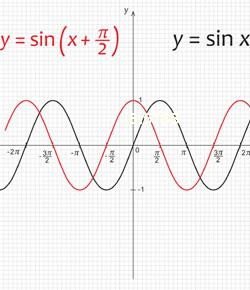# Area And Perimeter Of Rectangles, Triangles And Parallelograms Quiz

19 Questions | Total Attempts: 651SettingsAssesses and reinforces the student's understanding and ability to calculate areas and perimeters of fundamental shapes.

• 1.
The perimeter of a shape is
• A.

The distance around the outside edge

• B.

The space covered by the shape.

• C.

The length of all sides laid end to end

• 2.
The perimeter of an object is always the same as its area.
• A.

True

• B.

False

• 3.
What is the perimeter of this square?
• A.

10 cm

• B.

25 cm

• C.

20 cm

• D.

55 cm

• 4.
In square cm, what is the area of this square?
• 5.
What is the perimeter of this rectangle?
• A.

26 cm

• B.

13 cm

• C.

40 cm

• D.

58 cm

• 6.
What is the area of this rectangle?
• A.

26 cm²

• B.

13 cm²

• C.

58 cm²

• D.

40 cm²

• 7.
What is the length of side x?
• A.

275 cm

• B.

11 cm

• C.

5 cm

• 8.
What is the perimeter of this rectangle (include the unit)?
• 9.
What is the perimeter of this rectangle (include units)?
• 10.
What is the perimeter of this square?
• A.

12 cm

• B.

48 cm

• C.

576 cm

• D.

24 cm

• 11.
What is the area of this triangle (in square cm)?
• 12.
How long is the base of this triangle?
• 13.
Using Pythagoras, calculate the perimeter of this triangle (rounded to 1 dp):
• A.

8.5 cm

• B.

19.5 cm

• C.

11 cm

• D.

23

• 14.
In square cm, what is the area of this triangle?
• 15.
Using Pythagoras, what is the perimeter of this triangle (rounded to the nearest whole number)?
• 16.
What is the perimeter of this parallelogram?
• A.

19 cm

• B.

24 cm

• C.

30 cm

• D.

15 cm

• 17.
What is the area of this parallelogram?
• A.

16 cm²

• B.

28 cm²

• C.

19 cm²

• D.

32 cm²

• 18.
How long is the base of this parallelogram?
• 19.
What is the perimeter of this parallelogram?
Related Topics